This book is archived and will be removed July 6, 2022. Please use the updated version.

Process Costing

# 29 Explain and Compute Equivalent Units and Total Cost of Production in an Initial Processing Stage

As described previously, process costing can have more than one work in process account. Determining the value of the work in process inventory accounts is challenging because each product is at varying stages of completion and the computation needs to be done for each department. Trying to determine the value of those partial stages of completion requires application of the equivalent unit computation. The equivalent unit computation determines the number of units if each is manufactured in its entirety before manufacturing the next unit. For example, forty units that are 25% complete would be ten (40 × 25%) units that are totally complete.

Direct material is added in stages, such as the beginning, middle, or end of the process, while conversion costs are expensed evenly over the process. Often there is a different percentage of completion for materials than there is for labor. For example, if material is added at the beginning of the process, the forty units that are 100% complete with respect to material and 25% complete with respect to conversion costs would be the same as forty units of material and ten units (40 × 25%) completed with conversion costs.

For example, during the month of July, Rock City Percussion purchased raw material inventory of \$25,000 for the shaping department. Although each department tracks the direct material it uses in its own department, all material is held in the material storeroom. The inventory will be requisitioned for each department as needed.

During the month, Rock City Percussion’s shaping department requested \$10,179 in direct material and started into production 8,700 hickory drumsticks of size 5A. There was no beginning inventory in the shaping department, and 7,500 drumsticks were completed in that department and transferred to the finishing department. Wood is the only direct material in the shaping department, and it is added at the beginning of the process, so the work in process (WIP) is considered to be 100% complete with respect to direct materials. At the end of the month, the drumsticks still in the shaping department were estimated to be 35% complete with respect to conversion costs. All materials are added at the beginning of the shaping process. While beginning the size 5A drumsticks, the shaping department incurred these costs in July: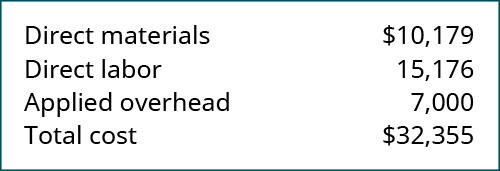These costs are then used to calculate the equivalent units and total production costs in a four-step process.

### Step One: Determining the Units to Which Costs Will Be Assigned

In addition to the equivalent units, it is necessary to track the units completed as well as the units remaining in ending inventory. A similar process is used to account for the costs completed and transferred. Reconciling the number of units and the costs is part of the process costing system. The reconciliation involves the total of beginning inventory and units started into production. This total is called “units to account for,” while the total of beginning inventory costs and costs added to production is called “costs to be accounted for.” Knowing the total units or costs to account for is helpful since it also equals the units or costs transferred out plus the amount remaining in ending inventory.

When the new batch of hickory sticks was started on July 1, Rock City Percussion did not have any beginning inventory and started 8,700 units, so the total number of units to account for in the reconciliation is 8,700: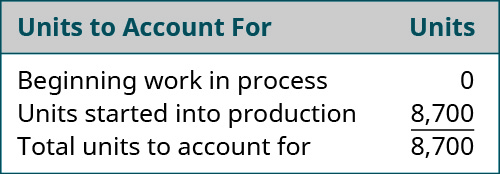The shaping department completed 7,500 units and transferred them to the testing and sorting department. No units were lost to spoilage, which consists of any units that are not fit for sale due to breakage or other imperfections. Since the maximum number of units that could possibly be completed is 8,700, the number of units in the shaping department’s ending inventory must be 1,200. The total of the 7,500 units completed and transferred out and the 1,200 units in ending inventory equal the 8,700 possible units in the shaping department.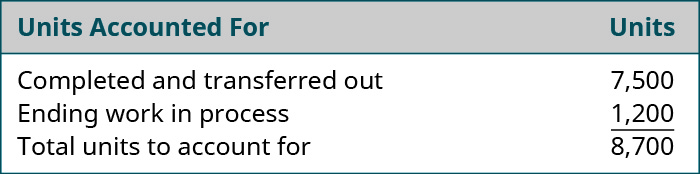### Step Two: Computing the Equivalent Units of Production

All of the materials have been added to the shaping department, but all of the conversion elements have not; the numbers of equivalent units for material costs and for conversion costs remaining in ending inventory are different. All of the units transferred to the next department must be 100% complete with regard to that department’s cost or they would not be transferred. So the number of units transferred is the same for material units and for conversion units. The process cost system must calculate the equivalent units of production for units completed (with respect to materials and conversion) and for ending work in process with respect to materials and conversion.

For the shaping department, the materials are 100% complete with regard to materials costs and 35% complete with regard to conversion costs. The 7,500 units completed and transferred out to the finishing department must be 100% complete with regard to materials and conversion, so they make up 7,500 (7,500 × 100%) units. The 1,200 ending work in process units are 100% complete with regard to material and have 1,200 (1,200 × 100%) equivalent units for material. The 1,200 ending work in process units are only 35% complete with regard to conversion costs and represent 420 (1,200 × 35%) equivalent units.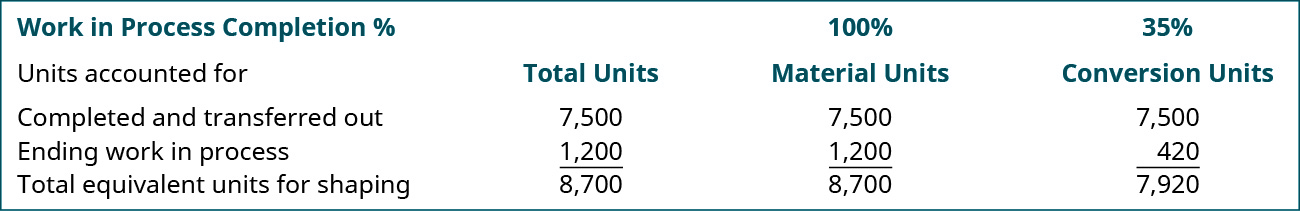### Step Three: Determining the Cost per Equivalent Unit

Once the equivalent units for materials and conversion are known, the cost per equivalent unit is computed in a similar manner as the units accounted for. The costs for material and conversion need to reconcile with the total beginning inventory and the costs incurred for the department during that month.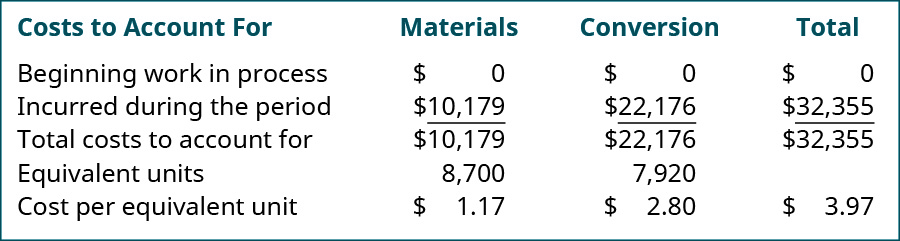The total materials costs for the period (including any beginning inventory costs) is computed and divided by the equivalent units for materials. The same process is then completed for the total conversion costs. The total of the cost per unit for material (\$1.17) and for conversion costs (\$2.80) is the total cost of each unit transferred to the finishing department (\$3.97).

### Step Four: Allocating the Costs to the Units Transferred Out and Partially Completed in the Shaping Department

Now you can determine the cost of the units transferred out and the cost of the units still in process in the shaping department. To calculate the goods transferred out, simply take the units transferred out times the sum of the two equivalent unit costs (materials and conversion) because all items transferred to the next department are complete with respect to materials and conversion, so each unit brings all its costs. But the ending WIP value is determined by taking the product of the work in process material units and the cost per equivalent unit for materials plus the product of the work in process conversion units and the cost per equivalent unit for conversion.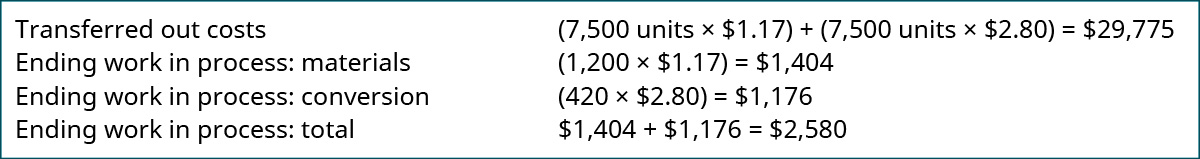This information is accumulated in a production cost report. This report shows the costs used in the preparation of a product, including the cost per unit for materials and conversion costs, and the amount of work in process and finished goods inventory. A complete production cost report for the shaping department is illustrated in (Figure).

Production Cost Report for the Shaping Department. (attribution: Copyright Rice University, OpenStax, under CC BY-NC-SA 4.0 license)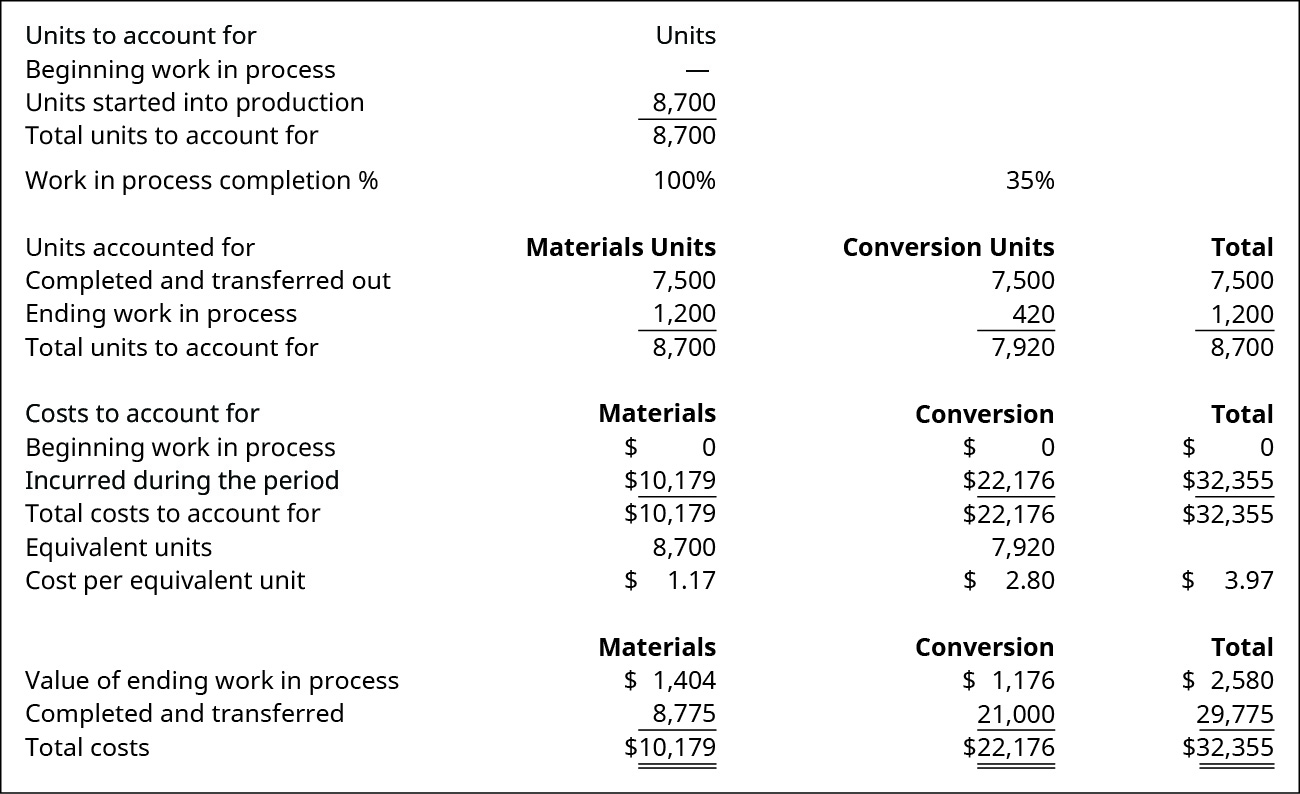Calculating Inventory Transferred and Work in Process Costs

Kyler Industries started a new batch of paint on October 1. The new batch consists of 8,700 cans of paint, of which 7,500 was completed and transferred to finished goods. During October, the manufacturing process recorded the following expenses: direct materials of \$10,353; direct labor of \$17,970; and applied overhead of \$9,000. The inventory still in process is 100% complete with respect to materials and 30% complete with respect to conversion. What is the cost of inventory transferred out and work in process? Assume that there is no beginning work in process inventory.

Solution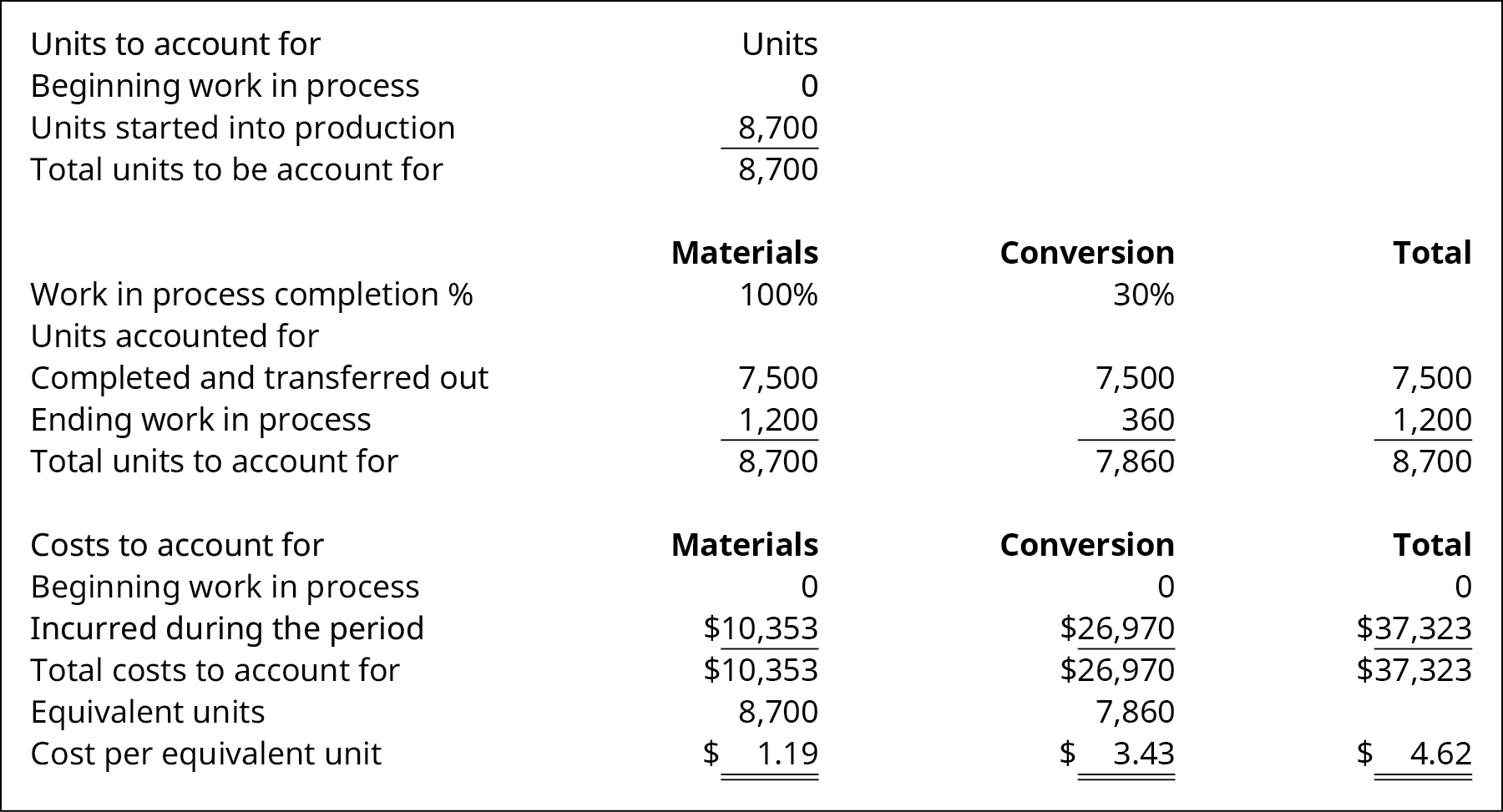### Key Concepts and Summary

• Process costing has a work in process inventory account for each department.
• Equivalent units of production for materials may differ from the equivalent units for conversion costs.
• The total units to account for is the number of units in the beginning work in process inventory plus the number of units started into production; this total also represents the sum of the number of units completed and the number of units in the ending work in process inventory.
• The cost per equivalent unit for materials is the total of the material costs for the beginning work in process inventory and the total of material costs incurred during the period.
• The cost per equivalent unit for conversion costs is the total of the conversion costs for the beginning work in process inventory and the total of conversion costs incurred during the period.
• The cost of units transferred to the next department is the number of units transferred times the total of the cost per equivalent unit of material plus the cost per equivalent unit for conversion costs.

(Figure)Direct material costs \$3 per unit, direct labor costs \$5 per unit, and overhead is applied at the rate of 100% of the direct labor cost. What is the value of the inventory transferred to the next department if beginning inventory was 2,000 units; 9,000 units were started; and 1,000 units were in ending inventory?

1. \$1,000
2. \$13,000
3. \$130,000
4. \$20,000

(Figure)Beginning inventory and direct material cost added during the month total \$55,000. What is the value of the ending work in process inventory if beginning inventory was 2,000 units; 9,000 units were started; and 1,000 units were in ending inventory?

1. \$1,000
2. \$5,000
3. \$50,000
4. \$55,000

B

(Figure)The initial processing department had a beginning inventory of 750 units and an ending inventory of 1,350 units, and it started 9,500 units into production. How many were transferred out to the next department?

1. 750
2. 1,350
3. 8,900
4. 10,250

(Figure)There were 1,000 units in ending inventory after transferring 16,000 units to finished goods inventory. If the beginning inventory was 2,000 units, how many units were started in process?

1. 1,000
2. 2,000
3. 15,000
4. 17,000

C

(Figure)What are the four steps involved in determining the cost of inventory transferred from one department to the next and the cost of work in process inventory?

Step 1: Determine the units to which costs are assigned. Step 2: Compute the equivalent units of production. Step 3: Determine the cost per equivalent unit. Step 4: Allocate the costs to the units transferred out and the units partially completed.

(Figure)What is the weighted-average method for computing the equivalent units of production?

(Figure)Given the following information, determine the equivalent units of ending work in process for materials and conversion under the weighted-average method:

• beginning inventory of 2,500 units is 100% complete with regard to materials and 60% complete with regard to conversion
• 18,000 units were started during the period
• 17,500 units were completed and transferred
• ending inventory is 100% complete with materials and 65% complete with conversion

(Figure)There were 1,700 units in beginning inventory that were 40% complete with regard to conversion. During the month, 8,550 units were started and 9,000 were transferred to finished goods. The ending work in process was 60% complete with regard to conversion costs, and materials are added at the beginning of the process. What is the total amount of equivalent units for materials and conversion at the end of the month using the weighted-average method?

(Figure)A company has 1,500 units in ending work in process that are 30% complete after transferring out 10,000 units. All materials are added at the beginning of the process. If the cost per unit is \$4 for materials and \$7 for conversion, what is the cost of units transferred out and in ending work in process inventory using the weighted-average method?

(Figure)There were 2,400 units in ending work in process inventory that were 100% complete with regard to material and 25% complete with regard to conversion costs. Ending work in process inventory had a cost of \$9,000 and a per-unit material cost of \$2. What was the conversion cost per unit using the weighted-average method?

(Figure)How many units must be in ending inventory if beginning inventory was 15,000 units, 55,000 units were started, and 57,000 units were completed and transferred out?

(Figure)How many units must have been completed and transferred if beginning inventory was 75,000 units, ending inventory was 72,000 units, and 290,000 units were started?

(Figure)Using the weighted-average method, compute the equivalent units of production if the beginning inventory consisted of 20,000 units; 55,000 units were started in production; and 57,000 units were completed and transferred to finished goods inventory. For this process, materials are added at the beginning of the process, and the units are 35% complete with respect to conversion.

(Figure)Using the weighted-average method, compute the equivalent units of production for a new company that started 85,000 units into production and transferred 67,000 to the second department. Assume that beginning inventory was 0. Conversion is considered to occur evenly throughout the process, while materials are added at the beginning of the process. The ending inventory for Equivalent Units: Conversion is 9,000 units.

(Figure)Mazomanie Farm completed 20,000 units during the quarter and has 2,500 units still in process. The units are 100% complete with regard to materials and 55% complete with regard to conversion costs. What are the equivalent units for materials and conversion?

(Figure)What are the total costs to account for if a company’s beginning inventory had \$231,432 in materials, \$186,450 in conversion costs, and added direct material costs (\$4,231,392), direct labor (\$2,313,392), and manufacturing overhead (\$1,156,696)?

(Figure)A company started the month with 8,329 units in work in process inventory. It started 23,142 units and had an ending inventory of 9,321. The units were 100% complete to materials and 67% complete with conversion. How many units were transferred out during the period?

(Figure)Given the following information, determine the equivalent units of ending work in process for materials and conversion using the weighted-average method:

• Beginning inventory of 750 units is 100% complete with regard to materials and 30% complete with regard to conversion.
• 9,500 units were started during the period.
• 8,900 units were completed and transferred.
• Ending inventory is 100% complete with regard to materials and 68% complete with regard to conversion.

(Figure)There were 2,000 units in beginning inventory that were 70% complete with regard to conversion. During the month, 15,000 units were started, and 16,000 were transferred to finished goods. The ending work in process was 55% complete with regard to conversion costs, and materials are added at the beginning of the process. What is the total amount of equivalent units for materials and conversion at the end of the month using the weighted-average method?

(Figure)A company has 100 units in ending work in process that are 40% complete after transferring out 750 units. If the cost per unit is \$5 for materials and \$2.50 for conversion, what is the cost of units transferred out and in ending work in process inventory using the weighted-average method?

(Figure)There were 1,500 units in ending work in process inventory that were 100% complete with regard to material and 60% complete with regard to conversion costs. Ending work in process inventory had a cost of \$7,200 and a per-unit material cost of \$3. What was the conversion cost per unit using the weighted-average method?

(Figure)Using the weighted-average method, compute the equivalent units of production if the beginning inventory consisted of 20,000 units, 55,000 units were started in production, and 57,000 units were completed and transferred to finished goods inventory. For this process, materials are 70% complete and the units are 30% complete with respect to conversion.

(Figure)What are the total costs to account for if a company’s beginning inventory had \$23,432 in materials and \$18,450 in conversion costs, and added direct material costs (\$41,392), direct labor (\$23,192), and manufacturing overhead (\$62,500)?

(Figure)A company started the month with 4,519 units in work in process inventory. It started 15,295 units and had an ending inventory of 4,936. The units were 100% complete to materials and 30% complete with conversion. How many units were transferred out during the period?

(Figure)Pant Risers manufactures bands for self-dressing assistive devices for mobility-impaired individuals. Manufacturing is a one-step process where the bands are cut and sewn. This is the information related to this year’s production: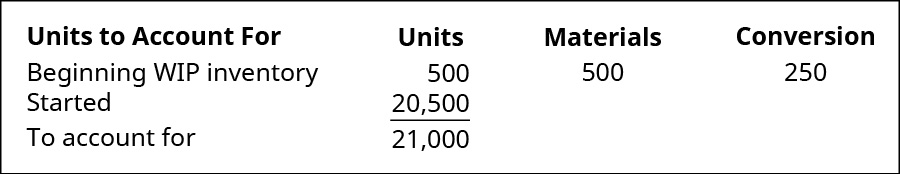Ending inventory was 100% complete as to materials and 70% complete as to conversion, and the total materials cost is \$57,540 and the total conversion cost is \$36,036. Using the weighted-average method, what are the unit costs if the company transferred out 17,000 units? What is the value of the inventory transferred out and the value of the ending WIP inventory?

(Figure)During March, the following costs were charged to the manufacturing department: \$14,886 for materials; \$14,656 for labor; and \$13,820 for manufacturing overhead. The records show that 30,680 units were completed and transferred, while 2,400 remained in ending inventory. There were 33,080 equivalent units of material and 31,640 of conversion costs. Using the weighted-average method, what is the cost of inventory transferred and the balance in work in process inventory?

(Figure)Materials are added at the beginning of a production process, and ending work in process inventory is 30% complete with respect to conversion costs. Use the information provided to complete a production cost report using the weighted-average method.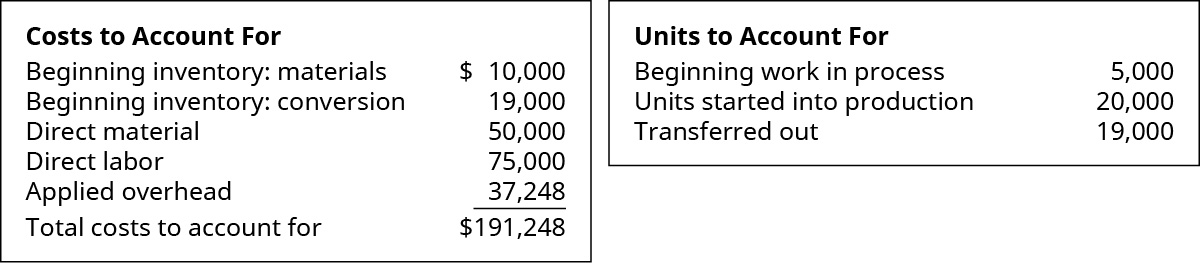(Figure)Narwhal Swimwear has a beginning work in process inventory of 13,500 units and transferred in 130,000 units before ending the month with 14,000 units that were 100% complete with regard to materials and 30% complete with regard to conversion costs. The cost per unit of material is \$5.80 and the cost per unit for conversion is \$8.20 per unit. Using the weighted-average method, what is the amount of material and conversion costs assigned to the department for the month?

(Figure)The following data show the units in beginning work in process inventory, the number of units started, the number of units transferred, and the percent completion of the ending work in process for conversion. Given that materials are added at the beginning of the process, what are the equivalent units for material and conversion costs for each quarter using the weighted-average method? Assume that the quarters are independent.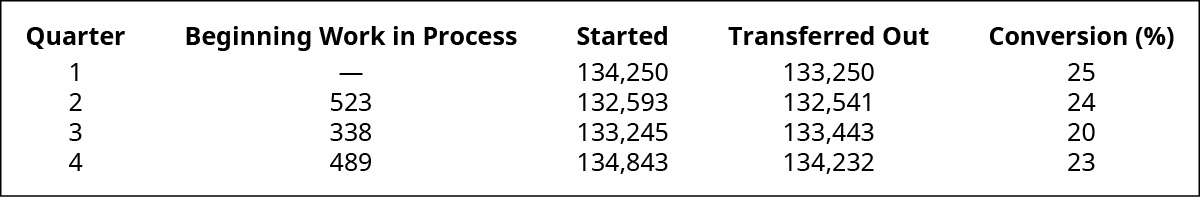(Figure)The following product costs are available for Stellis Company on the production of erasers: direct materials, \$22,000; direct labor, \$35,000; manufacturing overhead, \$17,500; selling expenses, \$17,600; and administrative expenses; \$13,400.

1. What are the prime costs?
2. What are the conversion costs?
3. What is the total product cost?
4. What is the total period cost?
5. If 13,750 equivalent units are produced, what is the equivalent material cost per unit?
6. If 17,500 equivalent units are produced, what is the equivalent conversion cost per unit?

(Figure)The following product costs are available for Kellee Company on the production of eyeglass frames: direct materials, \$32,125; direct labor, \$23.50; manufacturing overhead, applied at 225% of direct labor cost; selling expenses, \$22,225; and administrative expenses, \$31,125. The direct labor hours worked for the month are 3,200 hours.

1. What are the prime costs?
2. What are the conversion costs?
3. What is the total product cost?
4. What is the total period cost?
5. If 6,425 equivalent units are produced, what is the equivalent material cost per unit?
6. What is the equivalent conversion cost per unit?

(Figure)Vexar manufactures nails. Manufacturing is a one-step process where the nails are forged. This is the information related to this year’s production: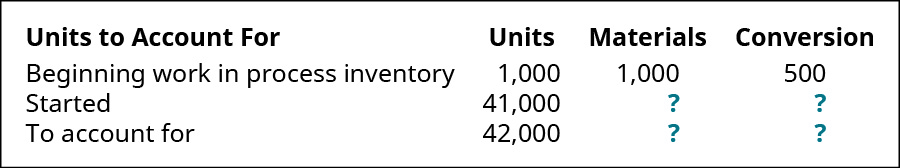Ending inventory was 100% complete as to materials and 70% complete as to conversion, and the total materials cost is \$115,080 and the total conversion cost is \$72,072. Using the weighted-average method, what are the unit costs if the company transferred out 34,000 units? Using the weighted-average method, prepare the company’s process cost summary for the month.

(Figure)During March, the following costs were charged to the manufacturing department: \$22,500 for materials; \$45,625 for labor; and \$50,000 for manufacturing overhead. The records show that 40,000 units were completed and transferred, while 10,000 remained in ending inventory. There were 45,000 equivalent units of material and 42,500 units of conversion costs. Using the weighted-average method, prepare the company’s process cost summary for the month.

(Figure)Ardt-Barger has a beginning work in process inventory of 5,500 units and transferred in 25,000 units before ending the month with 3,000 units that were 100% complete with regard to materials and 80% complete with regard to conversion costs. The cost per unit of material is \$5.45, and the cost per unit for conversion is \$6.20 per unit. Using the weighted-average method, prepare the company’s process cost summary for the month.

(Figure)The following data show the units in beginning work in process inventory, the number of units started, the number of units transferred, and the percent completion of the ending work in process for conversion. Given that materials are added at the beginning of the process, what are the equivalent units for material and conversion costs for each quarter using the weighted-average method? Assume that the quarters are independent.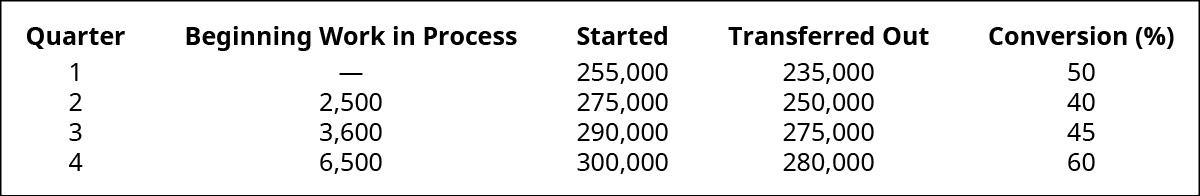(Figure)The following data show the units in beginning work in process inventory, the number of units started, the number of units transferred, and the percent completion of the ending work in process for conversion. Given that materials are added 50% at the beginning of the process and 50% at the end of the process, what are the equivalent units for material and conversion costs for each quarter using the weighted-average method? Assume that the quarters are independent.(Figure)The following data show the units in beginning work in process inventory, the number of units started, the number of units transferred, and the percent completion of the ending work in process for conversion. Given that materials are added 50% at the beginning of the process and 50% at the end of the process, what are the equivalent units for material and conversion costs for each quarter using the weighted-average method? Assume that the quarters are independent.### Glossary

production cost report
shows the costs used in the preparation of a product, including the cost per unit for materials and conversion costs and the amount of work in process and finished goods inventory
spoilage
any units that are not fit for sale due to breakage or other imperfections